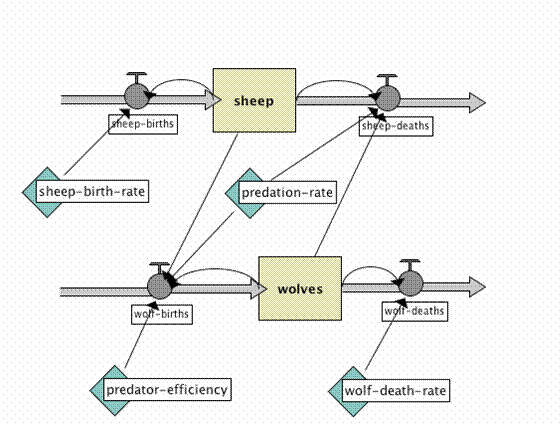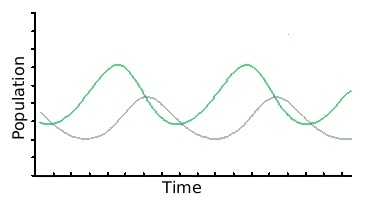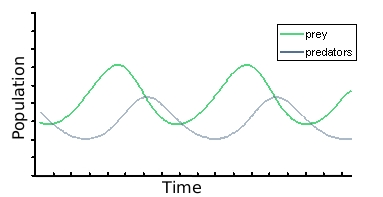Java Games: Flashcards, matching, concentration, and word search.# 2.2 Ecological Methods and Mathematical Models

AB
What are the three basic methods that ecologists use to study the environment?1) observing 2) experimenting 3) mathematical models
Which of the three basic methods that ecologists use to study the environment involves the use of computer simulations?mathematical models
Which of the three basic methods that ecologists use to study the environment involves the use of flowcharts like the one below?,mathematical models,Mathematical models use ____________ to show how one component in a system affects another.equations
What is the major advantage of using mathematical models to study the environment?They can be used to predict what will happen in the future.
What are some disadvantages of mathematically modeling relationships and populations in an ecosystem?1) require accurate data 2) make assumptions about the mathematical relationships between things in an ecosystem 3) Ecosystems are incredibly complex and hard to accurately model 4) It may take years of testing a model to see if it is predicting the future accurately
A graph like the one shown below probably represents the populations of a ______.,predator and its prey,Mr. McGee Truman High School

 This activity was created by a Quia Web subscriber. Learn more about QuiaCreate your own activities